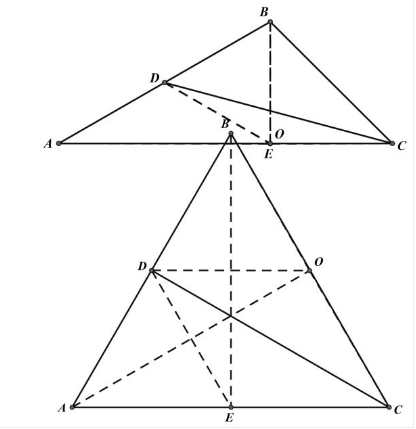【答案】 解: 作 $C D$ 的垂直平分线交 $A C$ 于 $E$

$\angle D E A=Q \angle D C E=\angle D A E$,
$\therefore A D=D E$, 又 $D$ 是 $A B$ 中点
$\therefore B E \perp A E$, 又 $\angle D C B=80^{\circ}$
$\triangle B D O$ 是等边三角形, 于是 $D O=B D$
$\angle B O A=90^{\circ}, \therefore A 、 B 、 O 、 E$ 四点共圆

$\therefore D O / / A C$, 且 $\angle D O A=30^{\circ}$
$\angle D A E=60^{\circ}, \triangle A D E$ 是等边三角形
$\therefore E$ 是 $A C$ 中点, $\therefore \triangle A B C$ 是等边三角形
$\therefore \angle A B C=60^{\circ}$